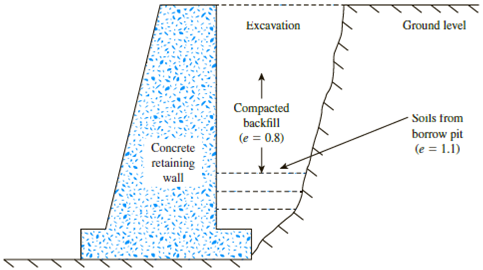Chapter 3, Problem 3.22PPrinciples of Geotechnical Enginee...

9th Edition
Braja M. Das + 1 other
ISBN: 9781305970939

Solutions

Chapter
SectionPrinciples of Geotechnical Enginee...

9th Edition
Braja M. Das + 1 other
ISBN: 9781305970939
Textbook Problem

Refer to Figure 3.14. After the construction of a concrete retaining wall, backfill material from a nearby borrow pit was brought into the excavation behind the wall and compacted to a final void ratio of 0.8. Given that the soil in the borrow pit has void ratio of 1.1, determine the volume of borrow material needed to construct 1 m3 of compacted backfill.Figure 3.14

To determine

Calculate the volume of borrow material needed to construct 1m3 of compacted backfill.

Explanation

Given information:

The void ratio of compacted backfill (ebackfill) is 0.8.

The void ratio of borrow pit (eborrow pit) is 1.1.

The volume of compacted backfill (V) is 1m3.

Calculation:

Calculate the dry unit weight (γd) using the relation.

γd=Gsγw1+e (1)

For compacted backfill,

Substitute 0.8 for e in Equation (1).

γdbackfill=Gsγw1+0.8=Gsγw1.8=0.556Gsγw

For borrow pit,

Substitute 1.1 for e in Equation (1).

γdborrow pit=Gsγw1+1.1=Gsγw2

Still sussing out bartleby?

Check out a sample textbook solution.

See a sample solution

The Solution to Your Study Problems

Bartleby provides explanations to thousands of textbook problems written by our experts, many with advanced degrees!

Get Started

Wheel A: 32A60H8V Wheel B: 39C100L6V Which wheel is harder?

Precision Machining Technology (MindTap Course List)

Explain the differences among data, information, and a database.

Database Systems: Design, Implementation, & Management

Calculate the reaction at D for the structure described in Prob. 5.11.

International Edition---engineering Mechanics: Statics, 4th Edition

Determine the maximum load in the weld in kips per inch of length.

Steel Design (Activate Learning with these NEW titles from Engineering!)